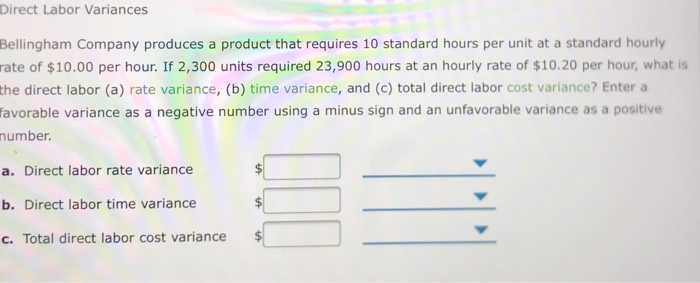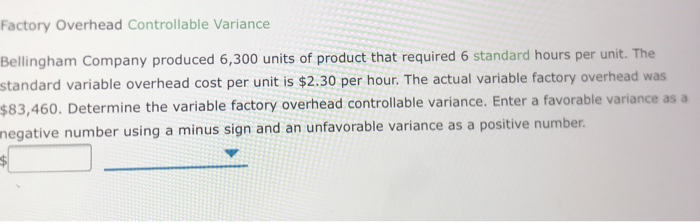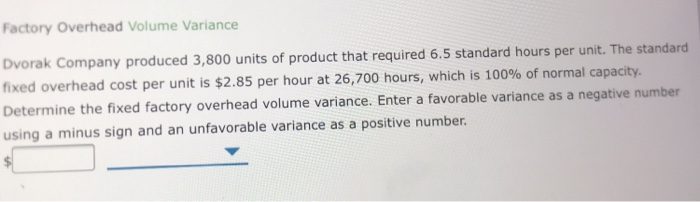# Solved: Direct Labor Variances Bellingham Company Produces A Product That Requires 10 Standard Hours Per Unit At A Standard Hourly Rate Of \$10.00 Per Hour. If 2,300 Units Required 23,900 Hours At An H

By |Direct Labor Variances Bellingham Company produces a product that requires 10 standard hours per unit at a standard hourly rate of \$10.00 per hour. If 2,300 units required 23,900 hours at an hourly rate of \$10.20 per hour, what is the direct labor (a) rate variance, (b) time variance, and (c) total direct labor cost variance? Enter a favorable variance as a negative number using a minus sign and an unfavorable variance as a positive mumber. a. Direct labor rate variance b. Direct labor time variance c. Total direct labor cost variance A Factory Overhead Controllable Variance Bellingham Company produced 6,300 units of product that required 6 standard hours per unit. The standard variable overhead cost per unit is \$2.30 per hour. The actual variable factory overhead was \$83,460. Determine the variable factory overhead controllable variance. Enter a favorable variance as a hegative number using a minus sign and an unfavorable variance as a positive number Factory Overhead Volume Variance Dvorak Company produced 3,800 units of product that required 6.5 standard hours per unit. The standard |fixed overhead cost per unit is \$2.85 per hour at 26,700 hours, which is 100% of normal capacity. Determine the fixed factory overhead volume variance. Enter a favorable variance as a negative number using a minus sign and an unfavorable variance as a positive number.